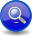#Boost C++ Libraries

...one of the most highly regarded and expertly designed C++ library projects in the world.

##### List Parser (`a % b`)
###### Description

The list operator, `a % b`, is a binary operator that matches a list of one or more repetitions of `a` separated by occurrences of `b`. This is equivalent to ```a >> *(b >> a)```.

###### Header
```// forwards to <boost/spirit/home/qi/operator/list.hpp>
#include <boost/spirit/include/qi_list.hpp>
```

Also, see Include Structure.

###### Model of

Notation

`a`, `b`
###### Expression Semantics

Semantics of an expression is defined only where it differs from, or is not defined in `BinaryParser`.

Expression

Semantics

```a % b```

Match a list of one or more repetitions of `a` separated by occurrences of `b`. This is equivalent to ```a >> *(omit[b] >> a)```.

NoteThe list operator `a % b` omits the attribute of parser `b`. If you need `b` to contribute to the result attribute choose ```a >> *(b >> a)``` over the list operator ```a % b```.
###### Attributes

Expression

Attribute

```a % b```

```a: A, b: B --> (a % b): vector<A>
a: Unused, b: B --> (a % b): Unused```

###### Complexity

The overall complexity of the List is defined by the complexity of its subject, `a`, multiplied by the number of repetitions. The complexity of the List itself is O(N), where N is the number successful repetitions.

###### Example
NoteThe test harness for the example(s) below is presented in the Basics Examples section.

Some using declarations:

```using boost::spirit::qi::int_;
```

Parse a comma separated list of numbers and put them in a vector:

```std::vector<int> attr;
test_phrase_parser_attr(
"111, 222, 333, 444, 555", int_ % ',', attr);
std::cout
<< attr << ',' << attr << ',' << attr << ','
<< attr << ',' << attr
<< std::endl;
```

 Copyright © 2001-2011 Joel de Guzman, Hartmut Kaiser Distributed under the Boost Software License, Version 1.0. (See accompanying file LICENSE_1_0.txt or copy at http://www.boost.org/LICENSE_1_0.txt)